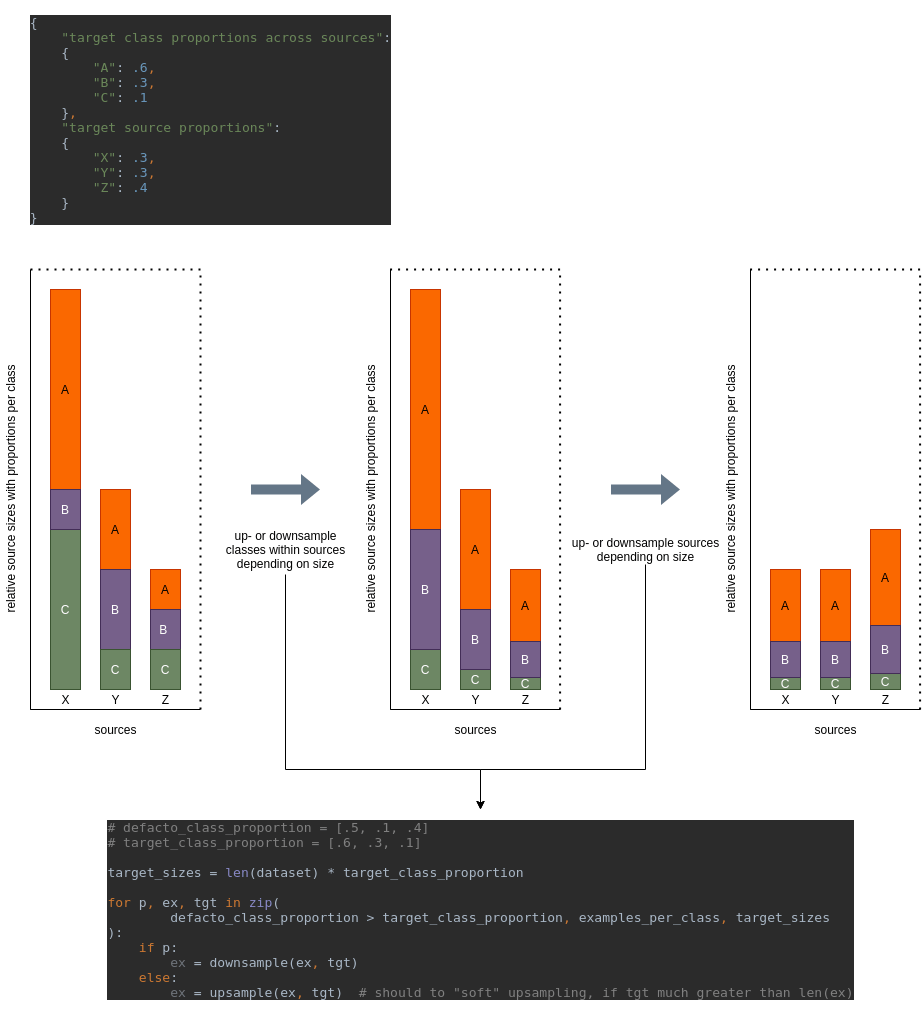# #StackBounty: #python #sampling #unbalanced-classes #mixture-distribution #resampling Resampling classes across weighted source distrib…

### Bounty: 50

I am sure this is a common problem, but googling only yielded false positives. I probably did not know what terms to search for. So here we go:

I have $$n$$ classes from $$m$$ different sources. Each source provides $$k leq n$$ of the different classes, and the probabilities of the $$k$$ classes vary between the sources. The examples of the classes also differ somewhat in their quality and overall nature across sources (in this case, images of certain entities). But one source alone does not provide sufficient numbers of examples. Hence, I need to combine the sources. But the sources are of varying representativeness for my target distribution $$T$$. $$T$$ is one of my sources as well. So I need to build some weighted combination of the sources whilst also accounting for their different probability distributions over the classes.

Are there any standard solutions to a problem like this? I assume so. I came up with the following:

1. Assign weights $$W_s in mathbf{R}^m$$ to sources
2. Define target class weights $$W_c in mathbf{R}^n$$ across sources
3. resample classes within sources to meet $$W_c$$
4. resample sources to meet $$W_s$$

The result is a weighted mixture distribution of the sources according to $$W_s$$ and $$W_c$$.

Can you help me with the following questions?

• Is my solution valid? Are there any statistical pitfalls that I overlooked?
• Are there better solutions?
• Are there (Python) packages that solve this more succinctly?
• What terms do I need to research to find more on this kind of problem?

Thanks!

Here is an example:For simplicity, I did not use the softening of `x ** .85` in the illustration.

``````import numpy as np
import pandas as pd

n = 10000
source_proportions = (props := [1, .5, .3])/np.sum(props)
examples_from_A = np.random.choice(["A", "B", "C"], int(n * source_proportions), p=(props := [.5, .1, .4])/np.sum(props))
examples_from_B = np.random.choice(["A", "B", "C"], int(n * source_proportions), p=(props := [.4, .4, .2])/np.sum(props))
examples_from_C = np.random.choice(["A", "B", "C"], int(n * source_proportions), p=(props := [1, 1, 1])/np.sum(props))

df = pd.DataFrame(
data={
"label": np.concatenate([examples_from_A, examples_from_B, examples_from_C]),
"source": ["X"]*len(examples_from_A)+["Y"]*len(examples_from_B)+["Z"]*len(examples_from_C)
}
)

# resample classes within sources
df = pd.concat([transform_class_frequencies(df[df.source == s], {"A": .6, "B": .3, "C": .1}, class_column="label") for s in df.source.unique()])
# resample sources
df = transform_class_frequencies(df, {"X": .3, "Y": .3, "Z": .4}, class_column="source")
``````

The resampling function I defined like this:

``````from functools import partial
from itertools import compress, starmap
from operator import itemgetter

import numpy as np
import pandas as pd
from sklearn.utils import resample

def downsample(klass, target_size, df, class_column="label"):
class_df = df[df[class_column] == klass]
n_examples = len(class_df)

assert target_size <= n_examples

surplus = (n_examples - target_size)
softened_surplus = np.ceil(surplus ** .85).astype(int)
target = n_examples - softened_surplus

downsampled_df = resample(class_df, replace=False, n_samples=target, random_state=42)
return downsampled_df

def upsample(klass, target_size, df, class_column="label"):
class_df = df[df[class_column] == klass]
n_examples = len(class_df)

assert target_size >= n_examples

missing = (target_size - n_examples)
softened_missing = np.ceil(missing ** .85).astype(int)

upsampled_df = resample(class_df, replace=True, n_samples=softened_missing, random_state=42)
return pd.concat([class_df, upsampled_df])

def transform_class_frequencies(df, class_2_target_class_proportion, class_column="label"):
"""Resamples classes from dataframe to reach a specified class frequency distributions.

Args:
df (pandas.DataFrame): Dataframe to resample classes from. Must have a column "label"
class_2_target_class_proportion (dict): mapping from class names to target proportions
"""
def normalize(a):
return a / np.sum(a)

df_classes, defacto_proportions = zip(*sorted(df[class_column].value_counts().to_dict().items(), key=itemgetter(0)))
class_2_target_class_proportion = {c: p for c, p in class_2_target_class_proportion.items() if c in df_classes}
classes, target_class_proportions = zip(*sorted(class_2_target_class_proportion.items(), key=itemgetter(0)))

target_class_proportions, defacto_proportions = map(normalize, (target_class_proportions, defacto_proportions))

target_sizes = np.ceil(len(df) * target_class_proportions).astype(int)

sampling = lambda sampling_function, sampling_mask: starmap(
partial(sampling_function, df=df, class_column=class_column),
)